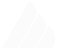# GyroDodge

## How it Works?

GyroDodge makes use of javascript's Device Orientation API to move the small white dot around your mobile screen & you can shoot projectiles at the direction you are heading toward by tapping on your screen. also also used p5js in gyrododge for making my life bit more easier

here's the code to get the device orientation of the device

``````// check if browser supports DeviceOrientationEvent event
if (!window.DeviceOrientationEvent) {
} else {
let alpha = event.alpha
let beta = event.beta
let gamma = event.gamma
})
}``````

to move the player in the desired location with these parameters, I add them to the player's `acceleration` by using p5.js's `Vector` class

check out the code

``````window.addEventListener("deviceorientation", e => {
this.tiltLR = e.gamma
this.tiltFB = e.beta
let gyro = createVector(this.tiltLR, this.tiltFB)
this.applyForce(gyro)
})``````

## How I created those asteroids?

To create those polygonal asteroids, I created a `Rock.js` class, which updates, renders, and generates them.

``````class Rock {
this.pos = createVector(x || random(width), y || random(height))
this.vel = p5.Vector.random2D()
this.vertices = []
this.offsets = []
this.total = random(5, 15)

for (let i = 0; i < this.total; i++) {
this.offsets[i] = random(-5, 5)
}
for (let i = 0; i < this.total; i++) {
let angle = map(i, 0, this.total, 0, TWO_PI)
let r = this.radius + this.offsets[i]
let x = r * cos(angle)
let y = r * sin(angle)
this.vertices.push({ x, y })
}
}
}``````

In the update method, I added the `velocity` to the `position` at each frame. I also checked for collision with the boundaries. If the rock hits the wall, then it just bounces back off them.

``````update() {

this.vel.x *= -1;
}
this.vel.y *= -1;
}
}``````

In render function, I just used p5js's `vertex` method to draw the polygonal shape

``````render() {
noFill();
stroke(255);
beginShape();
for (const v of this.vertices) {
vertex(this.pos.x + v.x, this.pos.y + v.y);
}
endShape(CLOSE);
}``````

In gyrodoge I also have a game mechanism where if you hit an asteroid it can break into multiple asteroids, here's how it works

``````breakup() {
let newA = [];
newA = new Rock(this.pos.x, this.pos.y, this.radius * 0.8);
newA = new Rock(this.pos.x, this.pos.y, this.radius * 0.8);
return newA;
}``````

And that's it, for the Rock.js class.

## Particles Class

I also added Particles class which emits particles in a random direction, and it extends the Bullet class

``````class Particle extends Bullet {
constructor(spos, angle) {
super(spos, angle)
this.vel = p5.Vector.random2D()
this.life = 1
}

render() {
this.vel.y += random(-0.2, 0.2)
push()
stroke(255, this.life * 255)
strokeWeight(2)
point(this.pos.x, this.pos.y)
pop()
}
die() {
this.life -= 0.03
}
}``````

## Bullet Class

check out the code

In bullet class, there's not much going on just basic stuff

``````class Bullet {
constructor(spos, angle) {
this.pos = createVector(spos.x, spos.y)
this.vel = p5.Vector.fromAngle(angle)
this.vel.mult(10)
this.angle = angle
}

update() {
}

render() {
...
}

// checks for hits
hits(target) {
var d = dist(this.pos.x, this.pos.y, target.pos.x, target.pos.y)
return true
}
return false
}

// if offscreen we will remove it from the bullet array
offscreen() {
if (this.pos.x > width || this.pos.x < 0) {
return true
}
if (this.pos.y > height || this.pos.y < 0) {
return true
}
return false
}
}``````

## Player Class

check out the code

In Player class we have some exciting stuff, let's see the constructor first

``````class Player {
constructor() {
this.pos = createVector(width / 2, height / 2);
this.acc = createVector(0, 0);
this.vel = createVector(0, 0);

this.tiltLR = 0;
this.tiltFB = 0;

this.isUsingKeyboard = false; // is using desktop?

if (!window.DeviceOrientationEvent) {
}

if (!('ontouchstart' in window)) {
this.isUsingKeyboard = true;
let pos = p5.Vector.sub(this.pos, createVector(e.offsetX, e.offsetY))
this.applyForce(pos)
})
} else {
this.tiltLR = e.gamma;
this.tiltFB = e.beta;
let gyro = createVector(this.tiltLR, this.tiltFB);
this.applyForce(gyro);
});
}
}``````

As you can see, I initialized the `deviceorientation` events and player's position and velocity stuff. The most basic method of the Player class is `collidePointPoly` which detects if a point hits a polygon.

``````collidePointPoly(px, py, target, vertices) {
var collision = false;
// go through each of the vertices, plus the next vertex in the list
var next = 0;
for (var current = 0; current < vertices.length; current++) {
// get next vertex in list if we've hit the end, wrap around to 0
next = current + 1;
if (next == vertices.length) next = 0;
// get the PVectors at our current position this makes our if statement a little cleaner
var vc = vertices[current];
var vn = vertices[next];
let vcy = target.pos.y + vc.y;
let vny = target.pos.y + vn.y;
let vcx = target.pos.x + vc.x;
let vnx = target.pos.x + vn.x;
if (((vcy > py && vny < py) || (vcy < py && vny > py)) &&
(px < (vnx - vcx) * (py - vcy) / (vny - vcy) + vcx)) {
collision = !collision;
}
}
return collision;
}``````

It's the vital part of the class because it checks if the Player hits any Asteroids (rock), and depending on that, it determines if the game is over or not.

## Game Class

check out the code

Game class is just a state manager for the game, which holds all the variables and state management.

we also have AssetMan class which preloads all the assets for the game (sound effects)

## Setting Up

And lastly but not least, we have index.js where I wrote all the necessary game logic and initialization.Made with love and gatsby by me